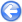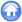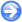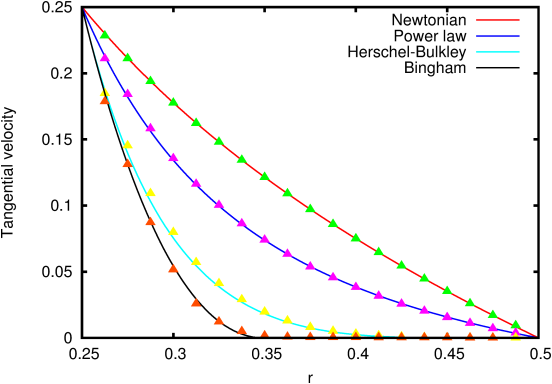### 6.3PASS: Creeping Couette flow of Generalised Newtonian fluids

Author
Stéphane Popinet
Command
sh couette.sh couette.gfs
Version
1.0.0
Required files
couette.sh profile prof-0.ref prof-1.ref prof-2.ref prof-3.ref
Running time
38 seconds

Following , we solve for the 2D creeping flow between two coaxial cylinders. The inner cylinder rotates at a constant speed. The outer cylinder is fixed. The viscosity is a function of the second principal invariant of the shear strain rate tensor:

|D|=
 √
 ∑ i,j
DijDij

where Dij=(∂iuj+∂jui)/2.

We use a general Herschel-Bulkley formulation of the form:

µ(|D|)=
 τy 2|D|
+µ|D|N−1,

where τy is the yield stress. The solutions obtained for the stationary tangential velocity profiles for Newtonian, Power law (N=0.5), Herschel-Bulkley (µ=0.0672, τy=0.12, N=0.5) and Bingham (µ=1, τy=10, N=1) fluids are illustrated on Figure 91, together with the analytical solutions given by .

The Bingham fluid case is a particularly severe test of the diffusion solver, as the outer part of the fluid ring (r>0.35) behaves likes a rigid body attached to the outer boundary.

 Figure 91: Tangential velocity as a function of radial position for various Generalised Newtonian fluids.